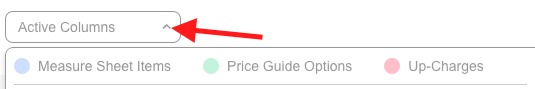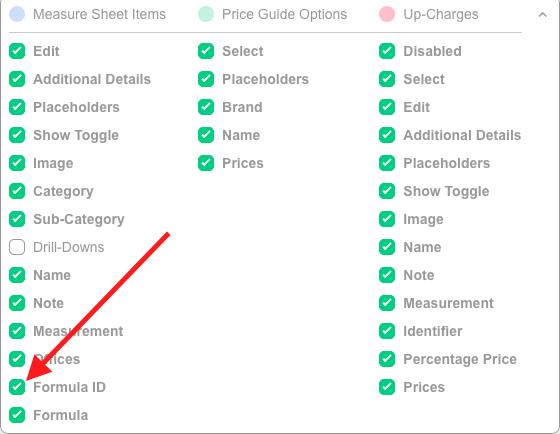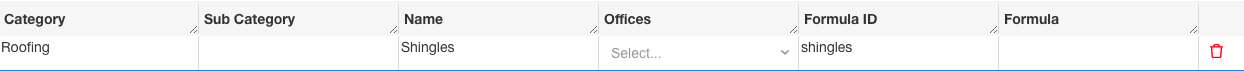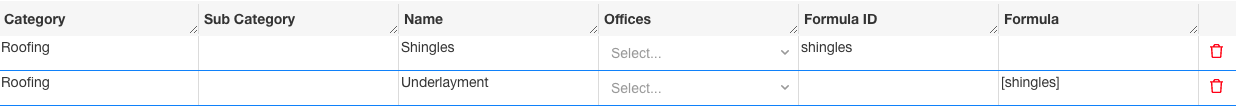# Formulas

Formulas allow you to perform math functions on measure sheet items. In addition, you can reference the values of other cells by using Formula IDs. Eagleview and Hover placeholders can be used in Formulas to automatically pull in measurements. To reference the value or quantity of another Measure Sheet Item you must first make up a Formula ID.
1. In the Price Guide click the gray drop-down menu on Active Columns to expand.2. Check off Formula ID and Formula under Measure Sheet Items.3. Close the Active Columns menu.
4. Create a Formula ID of your choice. In the example below we want to reference the value in Shingles. Type the word shingles into the Formula ID column of Shingles.5. Enter the Formula ID surrounded by square brackets [ & ] into the Formula of the Measure Sheet Item you wish to pull the value into. In this example we are pulling the quantity of Shingles into the quantity of Underlayment. Type [shingles] into the Formula column of Underlayment.6. List of Formula Functions
7. The numbers below under ‘Format/Syntax’ can be replaced with the Formula ID of a different Measure Sheet Item to pull in the value of that Measure Sheet Item. The below are examples to simply show the format of the command.
Function Format/Syntax
Subtract  – 
Multiply  * 
Divide  / 
Raise (To Power)  ** 
Square Root sqrt()
Round Up ceiling()
Round Down floor()
Average average({, , , , , , })
Sum sum({, , , , , , })
Count count({, , , , , , })
Min min({, , , , , , })
Max max({, , , , , , })
Random Number randomn(10)
Examples:1. In the Shingles Measure Sheet Item the EagleView Roof Area is being pulled in in square feet and divided by 100 to calculate the number of squares. It is then multiplied by 1.10 in order to add 10% waste. Note: The Shingles item has a Formula ID of SHINGLES.
2. The 15lbs Felt item uses the SHINGLES Formula ID which is then divided by 4 to output the number of rolls.
3. The RoofRunner item uses the SHINGLES Formula ID which is then divided by 10 to output the number of rolls.
4. The Install Labor item adds the SHINGLES and RIDGESHINGLES Formula IDs, divides them by 3 and uses the ceiling command to round up.